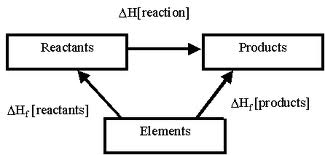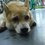# Type of Heat1)HESS'S LAW

Definition of Hess's law : states that the heat evolved or absorbed in a chemical process is the same whether the process takes place in one or in several steps. This is also known as the law of constant heat summation.

2)Heat of Combustion

Definition :Heat of formation is defined as the enthalpy change when one mole of a compound is formed from the elements in their stable states. For example, the heat of formation of water vapor is defined by the reaction:

Formula : Heat of reaction = [the sum of all heats of formation of all products] - [the sum of all heats of formation of all reactants]

3)Heat of Neutralization

Definition: Large concentrations of hydrogen ion and hydroxide ion cannot coexist in solution, because the neutralization reaction

H+ (aq) + OH- (aq) → H2O (l)

strongly favors the product (water).

4) Heat of Reaction

Definition ~ the heat evolved or absorbed during a chemical reaction taking place under conditions of constant temperature and of either constant volume or more often constant pressure

~ the quantity involved when gram equivalents of the substances enter into the reaction

5)Heat of Formation

Definition :the heat evolved or absorbed when one mole of a compound is formed from its constituent atomsNote by Nicole Ling
7 years, 4 months ago

This discussion board is a place to discuss our Daily Challenges and the math and science related to those challenges. Explanations are more than just a solution — they should explain the steps and thinking strategies that you used to obtain the solution. Comments should further the discussion of math and science.

When posting on Brilliant:

• Use the emojis to react to an explanation, whether you're congratulating a job well done , or just really confused .
• Ask specific questions about the challenge or the steps in somebody's explanation. Well-posed questions can add a lot to the discussion, but posting "I don't understand!" doesn't help anyone.
• Try to contribute something new to the discussion, whether it is an extension, generalization or other idea related to the challenge.

MarkdownAppears as
*italics* or _italics_ italics
**bold** or __bold__ bold
- bulleted- list
• bulleted
• list
1. numbered2. list
1. numbered
2. list
Note: you must add a full line of space before and after lists for them to show up correctly
paragraph 1paragraph 2

paragraph 1

paragraph 2

[example link](https://brilliant.org)example link
> This is a quote
This is a quote
    # I indented these lines
# 4 spaces, and now they show
# up as a code block.

print "hello world"
# I indented these lines
# 4 spaces, and now they show
# up as a code block.

print "hello world"
MathAppears as
Remember to wrap math in $$ ... $$ or $ ... $ to ensure proper formatting.
2 \times 3 $2 \times 3$
2^{34} $2^{34}$
a_{i-1} $a_{i-1}$
\frac{2}{3} $\frac{2}{3}$
\sqrt{2} $\sqrt{2}$
\sum_{i=1}^3 $\sum_{i=1}^3$
\sin \theta $\sin \theta$
\boxed{123} $\boxed{123}$